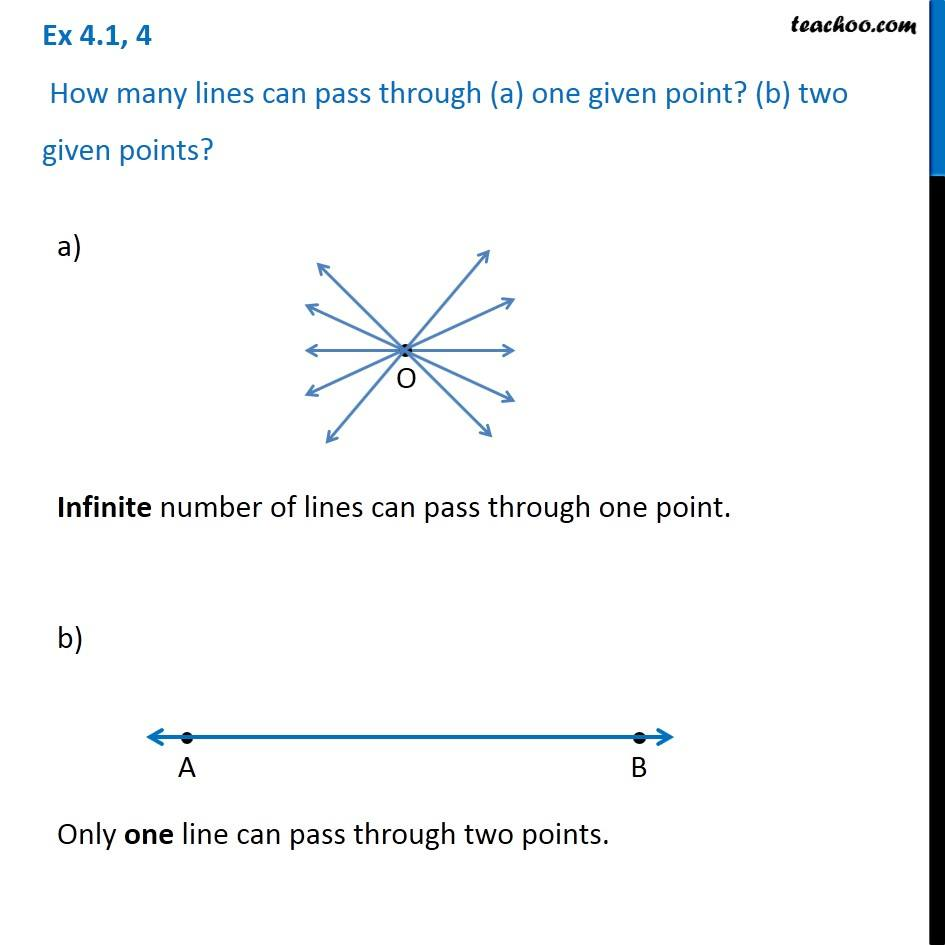1. Chapter 4 Class 6 Basic Geometrical Ideas
2. Serial order wise
3. Ex 4.1

Transcript

Ex 4.1, 4 How many lines can pass through (a) one given point? (b) two given points? a) Infinite number of lines can pass through one point. b) Only one line can pass through two points.

Ex 4.1

Chapter 4 Class 6 Basic Geometrical Ideas
Serial order wise